# Transimpedance Amplifier Noise Considerations

The LTC6268 and LTC6269 is a single/dual 500MHz FET-input operational amplifier with extremely low input bias current and low input capacitance. It also features low input referred current noise and voltage noise making it an ideal choice for high speed transimpedance amplifiers, CCD output buffers, and high-impedance sensor amplifiers. Its low distortion makes the LTC6268/LTC6269 an ideal amplifier for driving SAR ADCs.

Noise In Transimpedance Amplifiers

To minimize the LTC6268’s noise over a broad range of applications, careful consideration has been placed on input referred voltage noise (eN), input referred current noise (iN) and input capacitance CIN.

For a trans-impedance amplifier (TIA) application such as shown in Figure 1, all three of these op amp parameters, plus the value of feedback resistance RF, contribute to noise behavior in different ways, and external components and traces will add to CIN.

It is important to understand the impact of each parameter independently. Input referred voltage noise (eN) consists of flicker noise (or 1/f noise), which dominates at lower frequencies, and thermal noise which dominates at higher frequencies. For LTC6268, the 1/f corner, or transition between 1/f and thermal noise, is at 80kHz. The iN and RF contributions to input referred noise current at the minus input are relatively straight forward, while the eN contribution is amplified by the noise gain. Because there is no gain resistor, the noise gain is calculated using feedback resistor(RF) in conjunction with impedance of CIN as (1 + 2π RF • CIN • Freq), which increases with frequency. All of the contributions will be limited by the closed loop bandwidth. The equivalent input current noise is shown in Figures 2-5, where eN represents contribution from input referred voltage noise (eN), iN represents contribution from input referred current noise (iN), and RF represents contribution from feedback resistor (RF). TIA gain (RF) and capacitance at input (CIN) are also shown on each figure. Comparing Figures 2 & 3, and 4 & 5 for higher frequencies, eN dominates when CIN is high (5pF) due to the amplification mentioned above while iN dominates when CIN is low (1pF).Figure 2. Noise vs. Frequency CIN = 1pF, CF = 0.28pF, RF = 10kΩ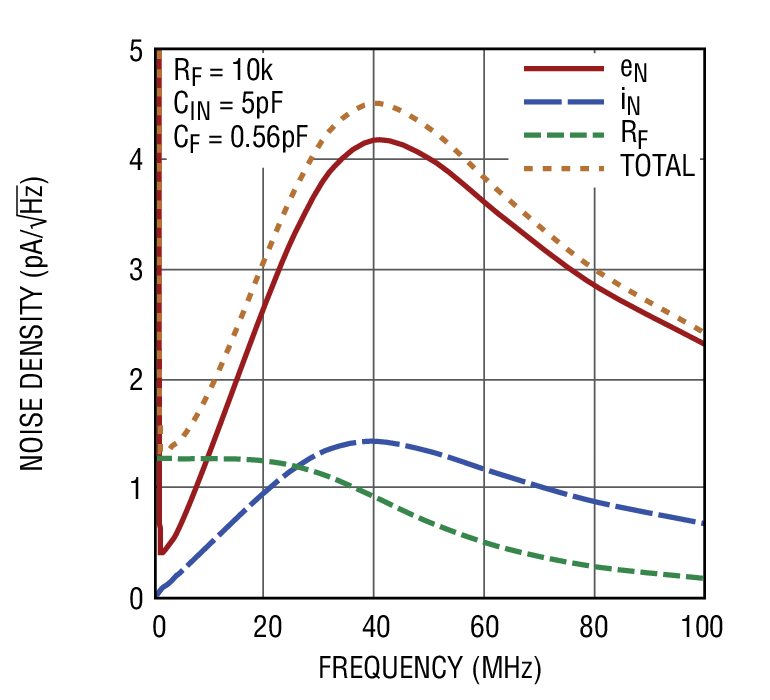Figure 3. Noise vs. Frequency CIN = 5pF, CF = 0.56pF, RF = 10kΩFigure 4. Noise vs. Frequency CIN = 1pF, CF = 0.08pF, RF =100kΩFigure 5. Noise vs. Frequency CIN = 5pF, CF = 0.18pF, RF = 100kΩ

At lower frequencies, the RF contribution dominates for 10k and 100k. Since wide band eN is 4.3nV/√Hz (see typical performance characteristics), RF contribution will become a lesser factor at lower frequencies if RF is less than 1.16kΩ as indicated by the following equation:Optimizing The Bandwidth For TIA Application

The capacitance at the inverting input node can cause amplifier stability problems if left unchecked. When the feedback around the op amp is resistive (RF), a pole will be created with RF ||CIN. This pole can create excessive phase shift and possibly oscillation. Referring to Figure 1, the response at the output is:Where RF is the DC gain of the TIA, ω is the natural frequency of the closed loop, which can be expressed as: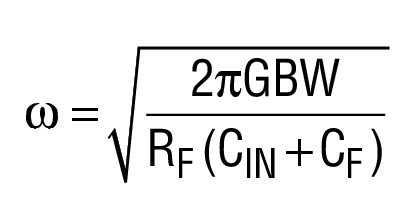ζ is the damping factor of the loop, which can be expressed asWhere CIN is the total capacitance at the inverting input node of the op amp, and GBW is the gain bandwidth of the op amp. There are two regions that the system will be stable regardless of CF. The first region is when RF is less than 1/(4π∙CIN∙GBW). In this region, the pole produced by the feedback resistor and CIN is at a high frequency which does not cause stability problems. The second region is where:Where AO is the DC open loop gain of the op amp, and the pole formed by RF CIN is the dominant pole.

For RF between these two regions, the small capacitor CF in parallel with RF can introduce enough damping to stabilize the loop. By assuming CIN >> CF, the following condition needs to be met for CF,The above condition implies that higher GBW will require lower feedback capacitance CF, which will have higher loop bandwidth. Table 1 shows the optimal CF for RF of 10kΩ and 100kΩ and CIN of 1pF and 5pF.

Achieving Higher Bandwidth With Higher Gain TIAs

Good layout practices are essential to achieving best results from a TIA circuit. The following two examples show drastically different results from an LTC6268 in a 499kΩ TIA. (See Figure 6.) The first example is with an 0603 resistor in a basic circuit layout. In a simple layout, without expending a lot of effort to reduce feedback capacitance, the bandwidth achieved is about 2.5MHz. In this case, the bandwidth of the TIA is limited not by the GBW of the LTC6268, but rather by the fact that the feedback capacitance is reducing the actual feedback impedance (the TIA gain itself) of the TIA. Basically, it’s a resistor bandwidth limitation. The impedance of the 499kΩ is being reduced by its own parasitic capacitance at high frequency. From the 2.5MHz bandwidth and the 499kΩ low frequency gain, we can estimate the total feedback capacitance as C = 1/(2π • 2.5MHz • 499kΩ) = 0.13pF. That’s fairly low, but it can be reduced further.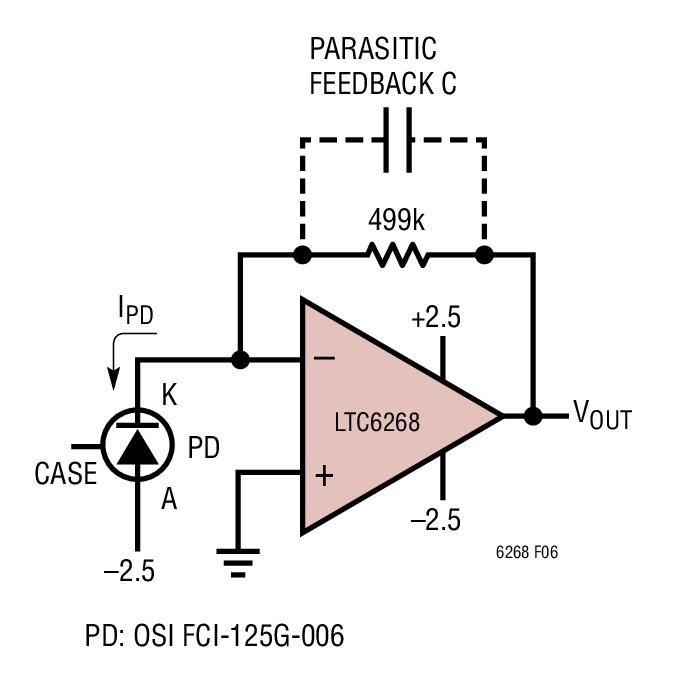Figure 6. LTC6268 and Low Capacitance Photodiode in a 499kΩ TIAFigure 7. Frequency Response of 499kΩ TIA without Extra Effort to Reduce Feedback Capacitance is 2.5MHz

With some extra layout techniques to reduce feedback capacitance, the bandwidth can be increased. Note that we are increasing the effective “bandwidth” of the 499kΩ resistance. One of the main ways to reduce capacitance is to increase the distance between the plates, in this case the plates being the two endcaps of the component resistor. For that reason, it will serve our purposes to go to a longer resistor. An 0805 is longer than an 0603, but its endcaps are also larger in area, increasing capacitance again. However, increasing distance between the endcaps is not the only way to decrease capacitance, and the extra distance between the resistor endcaps also allows the easy application of another technique to reduce feedback capacitance. A very powerful method to reduce plate to plate capacitance is to shield the E field paths that give rise to the capacitance. In this particular case, the method is to place a short ground trace between the resistor pads, near the TIA output end.

Such a ground trace shields the output field from getting to the summing node end of the resistor and effectively shunts the field to ground instead. Keeping the trace close to the output end increases the output load capacitance very slightly. See Figure 8 for a pictorial representation.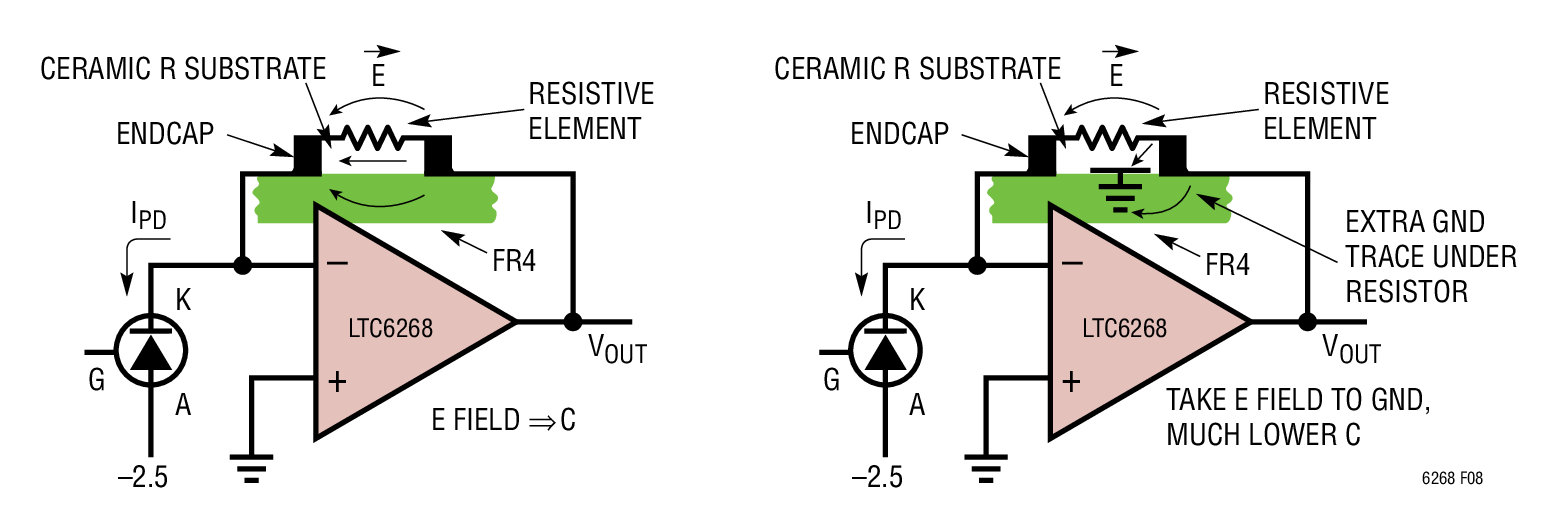Figure 8. A Normal Layout at Left and a Field-Shunting Layout at Right. Simply Adding a Ground Trace Under the Feedback Resistor Does Much to Shunt Field Away from the Feedback Side and Dumps It to Ground. Note That the Dielectric Constant of Fr4 and Ceramic Is Typically 4, so Most of the Capacitance Is in the Solids and Not Through the Air. (Reduced Pad Size On Right Is Not Shown.

Figure 9 shows the dramatic increase in bandwidth simply by careful attention to low capacitance methods around the feedback resistance. Bandwidth was raised from 2.5MHz to 11.2MHz, a factor greater than 4. Methods implemented were two:

1. Minimal pad sizing. Check with your board assembler for minimum acceptable pad sizing, or assemble this resistor using other means, and
2. Shield the feedback capacitance using a ground trace under the feedback resistor near the output side.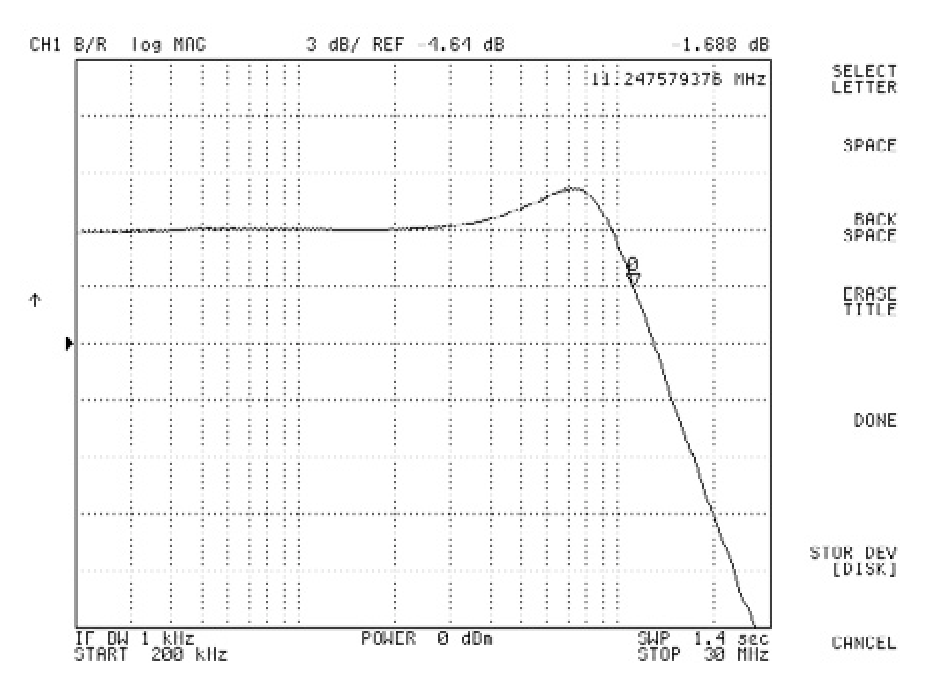Figure 9. LTC6268 in a 499kΩ TIA with extra Layout Effort to Reduce Feedback Capacitance Achieves 11.2MHz BW